# HBSE 8th Class Maths Solutions Chapter 15 Introduction to Graphs Ex 15.3

Haryana State Board HBSE 8th Class Maths Solutions Chapter 15 Introduction to Graphs Ex 15.3 Textbook Exercise Questions and Answers.

## Haryana Board 8th Class Maths Solutions Chapter 15 Introduction to Graphs Exercise 15.3

Question 1.
Draw the graphs for the following tables of values, with suitable scales on the axes.
(a) Cost of apples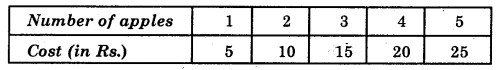(b) Distance travelled by a car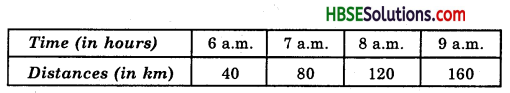(i) How much distance did the car cover during the period 7.30 a.m. to 8 a.m. ?
(ii) What was the time when the car had covered a distance of 100 km since it’s start ?
(c) Interest on deposits for a year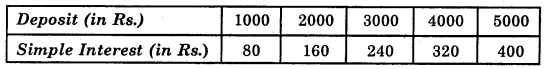(i) Does the graph pass through the origin ?
(ii) Use the graph to find the interest on Rs. 2500 for a year.
(iii) To get an interest of Rs. 280 per year, how much money should be deposited ?
Solution:
(a)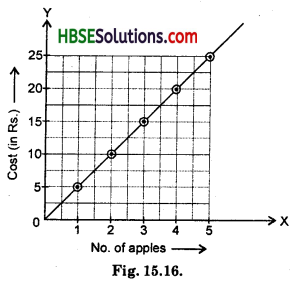Scale : Horizontal: 1 unit = 1 apple
Vertical: 1 unit = Rs. 5
Here, 10 small division = 1 unit
(i) Mark no. of apples along horizontal axis.
(ii) Mark cost (in Rs.) along vertical axis.
(iii) Plot the points as shown in graph.
(iv) Join the points (1, 5), (2, 10), (3, 15), (4, 20) and (5, 25).(b)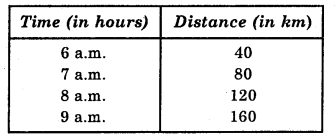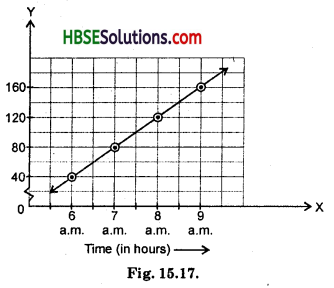Scale : 2 units = 1 hour (Horizontal line)
1 unit = 40 km (Vertical line)
(i) Corresponding to 7.30 a.m. on horizontal axis, we get the distance 100 km on vertical axis.
So, distance covered by car during 7.30 a.m. and 8 a.m. = 120 – 100 = 20 km.
(ii) 7.30 a.m.

(c)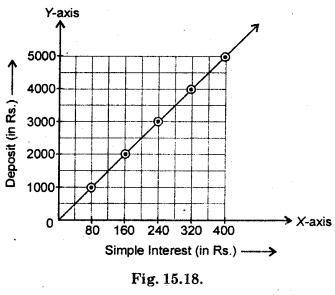Scale :
Horizontal : 1 unit = Rs. 80
Vertical: 1 unit = Rs. 1000
Hence, 10 small division = 1 unit.
(i) Mark simple interest (in Rs.) along horizontal axis.
(ii) Mark deposite (in Rs.) along vertical axis
(iii) Plot the points as showin in graph.
(iv) Joint the points (80, 1000), (160, 2000), (240, 3000), (320, 4000), (400, 5000)Question 2.
Draw a graph for the following :
(i)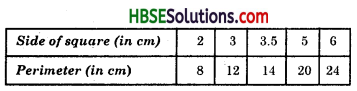Is it a linear graph ?
(ii)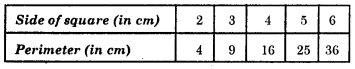Is it a linear graph ?
Solution: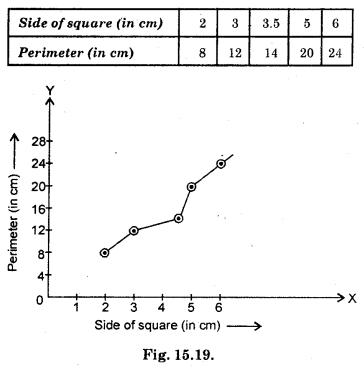Scale : 1 unit on horizontal line = 1 cm
1 unit on vertical line = 4 cm
Since graph is not a straight line.
∴ It is not a linear graph.
(ii)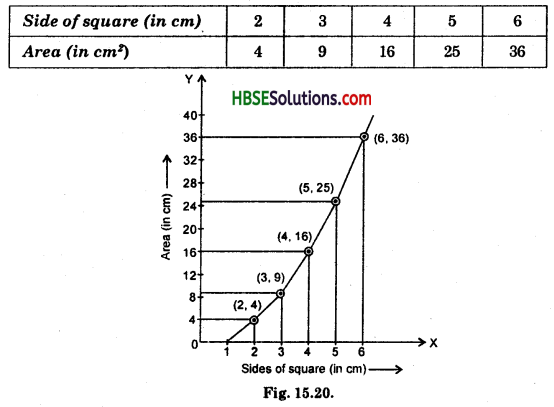Scale : 1 unit on X-axis = 1 cm
1 unit on Y-axis = 4 cm2